## Suppose light from a 632.8 nm helium-neon laser shines through a diffraction grating ruled at 520 lines/mm. How many bright lines are formed

Question

Suppose light from a 632.8 nm helium-neon laser shines through a diffraction grating ruled at 520 lines/mm. How many bright lines are formed on a screen a distance away?

in progress 0
6 months 2021-07-16T11:52:12+00:00 1 Answers 0 views 0

1 bright fringe every 33 cm.

Explanation:

The formula to calculate the position of the m-th order brigh line (constructive interference) produced by diffraction of light through a diffraction grating is: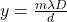where

m is the order of the maximum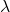is the wavelength of the light

D is the distance of the screen

d is the separation between two adjacent slit

Here we have: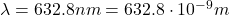is the wavelength of the light

D = 1 m is the distance of the screen (not given in the problem, so we assume it to be 1 meter)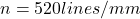is the number of lines per mm, so the spacing between two lines is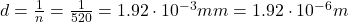Therefore, substituting m = 1, we find: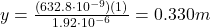So, on the distant screen, there is 1 bright fringe every 33 cm.